• 计算给定起点和终点的条线段之间的最短距离。 改编在 Dan Sunday 网站上找到的算法 ( http://softsurfer.com/Archive/algorithm_0106/algorithm_0106.htm#dist3D_Segment_to_Segment ）。 用法：输入条线段的...matlab
• 一个向量有个属性，大小和方向，我们先确定u’的大小（即长度，或者模），从u的末端做v的垂线，那么d就是u’的长度。而u’和v的方向是相同的，v的方向v/|v|也就是u’的方向。所以有 ...

向量投影

给定一个向量u和v，求u在v上的投影向量，如下图。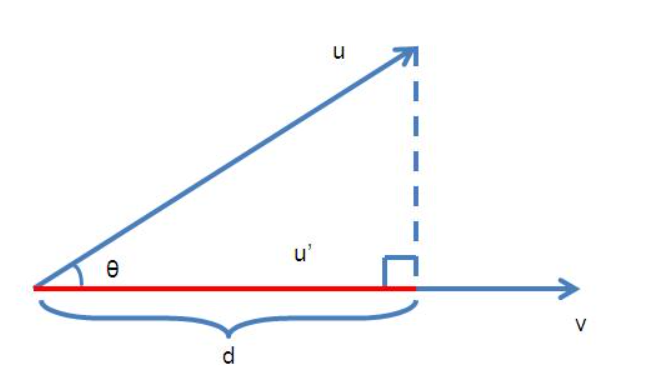假设u在v上的投影向量是u’，且向量u和v的夹角为theta。一个向量有两个属性，大小和方向，我们先确定u’的大小（即长度，或者模），从u的末端做v的垂线，那么d就是u’的长度。而u’和v的方向是相同的，v的方向v/|v|也就是u’的方向。所以有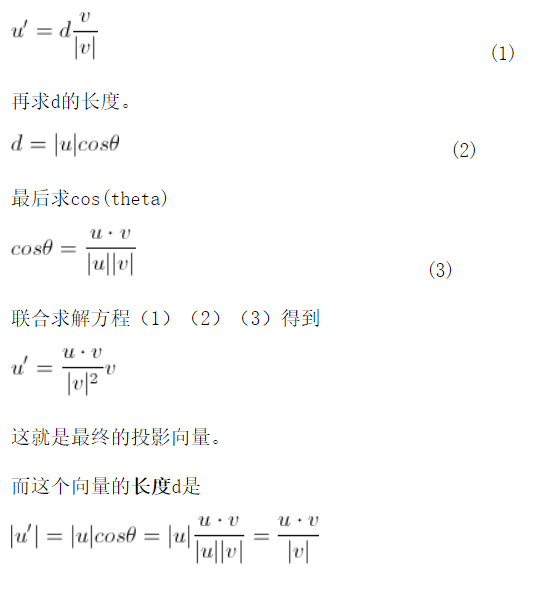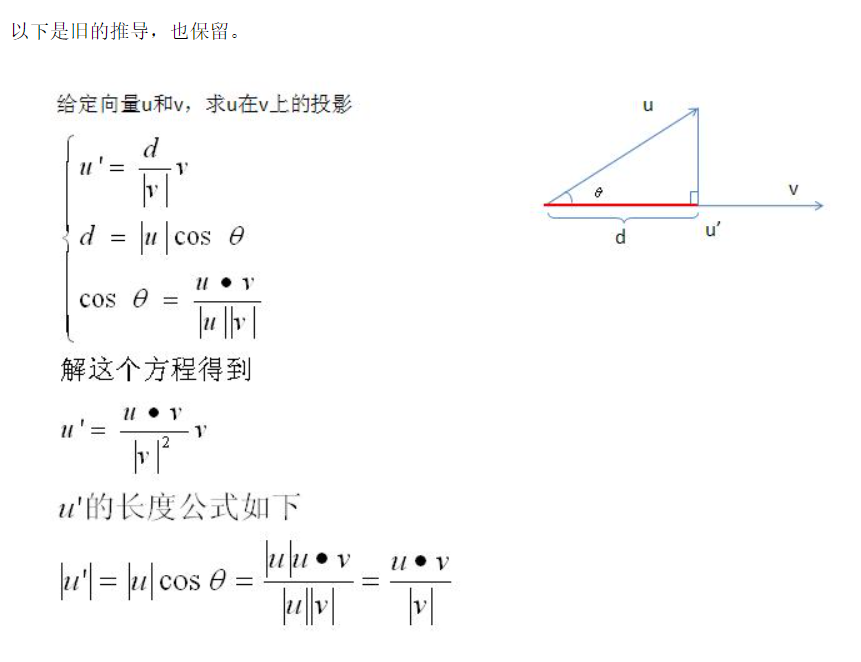// 计算点到直线的距离
// 返回值：点C到直线AB的最近距离；nearestPoint是直线AB上距离点C最近的点
template <class T>
float MyPointToLine(const T &C, const T &A, const T &B, T &nearestPoint)
{
float minDistance=0;
T ab = B-A;// 直线AB方向向量
T ac = C-A;
T n1 = ac^ab;//ac叉乘ab，得到平面ABC的法向量
T n2 = ab^n1;//ab叉乘n1，得到平行于平面ABC且垂直AB的向量
n2.normalize();//单位化
minDistance = ac*n2;//AC在n2方向上的投影（投影就是点C到直线AB的最近距离）
nearestPoint = C-n2*minDistance;
return minDistance;
}

// 求一个点到线段的最近距离。算法来自于网络。
// 返回值：点C到线段AB的最近距离；nearestPoint是线段AB上距离点C最近的点
template <class T>
float MyPointToLineSegment(const T &C, const T &A, const T &B, T &nearestPoint)
{
T ac = C-A;
T ab = B-A;//线段所在直线向量

float f = ac*ab;
if ( f<0 )//C点在AB上的投影位于A的左侧    不在线段AB的范围内
{
nearestPoint = A;
return ac.length();
}
else if (f>ab*ab)//C点在AB上的投影位于B的右侧     不在线段AB的范围内
{
nearestPoint = B;
return (B-C).length();
}
else//C点在AB上的投影位于线段AB内部
{
float abLen2 = ab.length2();//ab长度的平方
nearestPoint = A;
float epsilon = 0.000001f;
if ( abLen2 - 0.0f > epsilon )//ab长度不等于0，亦即A、B两点不重合
{
nearestPoint += ab*(f/abLen2);
}
return (nearestPoint-C).length();
}
}
// 计算两条直线之间的距离
// E是直线AB上一点，F是直线CD上一点，线段EF是直线AB到直线CD距离最短的线段
// 返回值为线段EF的长度
template <class T>
float MyLineToLine(const T& A, const T &B, const T &C, const T &D, T &E, T &F)
{
// 设CD在过AB且平行CD的平面π上的投影为C'D'，M、N为CD上两点，P、Q为C'D'上两点，
// AM⊥CD，AP⊥C'D'，BN⊥CD，BQ⊥C'D'，可以证明P、Q分别为M、N在π上的投影
// 并且有△AEP∽△BEQ，所以AE/EB=AP/BQ，计算出AP和BQ的长度即可
float minDistance=0;
T ab = B-A;
T cd = D-C;
T n = ab^cd;//叉乘。直线ab和cd公共垂直的直线方向向量
float epsilon = 1e-6;
if (n.length()>epsilon)//ab和cd不平行
{
n.normalize();//单位化n
minDistance = n*(C-A);
}
return minDistance;
}

展开全文c++
• 本文利用解析方法，求出了空间两线段的最短距离，文末附上了matlab程序。方法简单，可靠，程序复制粘贴后即可使用。良心之作！ 求空间两线段的最短距离和求空间两直线的最短距离不同，直线可以无限延伸，而线段不能...1 思路

求空间两线段的最短距离和求空间两直线的最短距离不同，直线可以无限延伸，而线段不能。
设空间中有两条线段 AB和 CD，设A点的坐标为 ( x 1 , y 1 , z 1 ) (x_1,y_1,z_1) ，B点的坐标为 ( x 2 , y 2 , z 2 ) (x_2,y_2,z_2) , C点的坐标为 ( x 3 , y 3 , z 3 ) (x_3,y_3,z_3) ，D点的坐标为 ( x 4 , y 4 , z 4 ) (x_4,y_4,z_4)

设P是直线AB上的一点，想象P点可以在直线AB上滑动，采用参数方程描述P点坐标。设P点坐标为 ( X , Y , Z ) (X,Y,Z) ，则：
{ X = x 1 + s ( x 2 − x 1 ) Y = y 1 + s ( y 2 − y 1 ) Z = z 1 + s ( z 2 − z 1 ) \left\{\begin{array}{l} X=x_{1}+s\left(x_{2}-x_{1}\right) \\ Y=y_{1}+s\left(y_{2}-y_{1}\right) \\ Z=z_{1}+s\left(z_{2}-z_{1}\right) \end{array}\right.
当 0 ≤ s ≤ 1 0 \leq s \leq 1 时，P是线段AB上的点；当 s < 0 s<0 时，P是BA延迟线上的点；当 s > 0 s>0 时，P是AB延迟线上的点；

同理可以设Q是直线CD上的点，设Q点坐标为 ( U , V , W ) (U,V,W) ，则
{ U = x 3 + t ( x 4 − x 3 ) V = y 3 + t ( y 4 − y 3 ) W = z 3 + t ( z 4 − z 3 ) \left\{\begin{array}{l} U=x_{3}+t\left(x_{4}-x_{3}\right) \\ V=y_{3}+t\left(y_{4}-y_{3}\right) \\ W=z_{3}+t\left(z_{4}-z_{3}\right) \end{array}\right.
当 0 ≤ t ≤ 1 0 \leq t \leq 1 时，Q是线段CD上的点；当 t < 0 t<0 时，Q是DC延迟线上的点；当 t > 0 t>0 时，Q是CD延迟线上的点；

那么P,Q之间的距离可以表示为：
P Q = ( X − U ) 2 + ( Y − V ) 2 + ( Z − W ) 2 P Q=\sqrt{(X-U)^{2}+(Y-V)^{2}+(Z-W)^{2}}

求两直线的最短距离，就是求PQ的最短距离。我们可以令：
f ( s , t ) = P Q 2 = ( X − U ) 2 + ( Y − V ) 2 + ( Z − W ) 2 f(s, t)=P Q^{2}=(X-U)^{2}+(Y-V)^{2}+(Z-W)^{2}
这样就把问题转为成了求 f ( s , t ) f(s, t) 最小值的问题，我们知道函数极值问题可以通过求导，令导数为0的方法解决，对多元函数也是一样。
对函数 f ( s , t ) f(s, t) 求关于 s , t s,t 的偏导数，令偏导数为0，可以得到一个二元一次方程组。
{ ∂ f ( s , t ) ∂ s = 0 ∂ f ( s , t ) ∂ t = 0 \left\{\begin{array}{l} \frac{\partial f(s, t)}{\partial s}=0 \\ \frac{\partial f(s, t)}{\partial t}=0 \end{array}\right.
求解这个方程得到 s , t s,t 的值，若 s , t s,t 满足， 0 ≤ s ≤ 1 0 \leq s \leq 1 0 ≤ t ≤ 1 0 \leq t \leq 1 ,则P在线段AB上，Q在线段CD上。

若 s , t s,t 不满足， 0 ≤ s ≤ 1 0 \leq s \leq 1 0 ≤ t ≤ 1 0 \leq t \leq 1 时，举例说明。比如 s > 1 s>1 ，可以将问题转为求 s = 1 s=1 时的P点到线段CD最短距离(这里大家想一想就明白了)。
求点到线段的最短距离原理和上文类似。还是设Q为直线CD上的点，Q点坐标为 ( U , V , W ) (U,V,W) ，则
{ U = x 3 + t ( x 4 − x 3 ) V = y 3 + t ( y 4 − y 3 ) W = z 3 + t ( z 4 − z 3 ) \left\{\begin{array}{l} U=x_{3}+t\left(x_{4}-x_{3}\right) \\ V=y_{3}+t\left(y_{4}-y_{3}\right) \\ W=z_{3}+t\left(z_{4}-z_{3}\right) \end{array}\right.
求点到线段的最短距离，还是求PQ的最短距离，但此时只有一个变量 t t ,我们可以令：
f ( t ) = P Q 2 = ( X − U ) 2 + ( Y − V ) 2 + ( Z − W ) 2 f(t)=P Q^{2}=(X-U)^{2}+(Y-V)^{2}+(Z-W)^{2}
对 f ( t ) f(t) 关于 t t 求导，并令导数等于0，求出 t t 。然后对 t t 进行判断就可以了。若 t > 1 t>1 ，令 t = 1 t=1 ；若 t < 0 t<0 ，令 t = 0 t=0 。最后我们将 s , t s,t 的值代入
P Q = ( X − U ) 2 + ( Y − V ) 2 + ( Z − W ) 2 P Q=\sqrt{(X-U)^{2}+(Y-V)^{2}+(Z-W)^{2}}
就完事了！

2 matlab代码

对大家有帮助的话记得点个赞，收藏一下！！

主函数

%main.m
A=[0 3 0];
B=[3 3 0];
C=[2 5 2];
D=[5 5 4];
%dis为线段AB、CD最短距离
[dis,P1,Q1,P2,Q2]=segmentDistance(A,B,C,D);
%画线段AB
plot3([A(1),B(1)],[A(2),B(2)],[A(3),B(3)],'r','LineWidth',2)
hold on
%画线段CD
plot3([C(1),D(1)],[C(2),D(2)],[C(3),D(3)],'b','LineWidth',2)
hold on
%画直线AB、CD最短距离
plot3([P1(1),Q1(1)],[P1(2),Q1(2)],[P1(3),Q1(3)],'k--','LineWidth',1.5)
hold on
%画线段AB、CD最短距离
plot3([P2(1),Q2(1)],[P2(2),Q2(2)],[P2(3),Q2(3)],'c','LineWidth',1)
xlabel('X')
ylabel('Y')
zlabel('Z')
grid on

两线段最短距离函数

function [dis,P1,Q1,P2,Q2]=segmentDistance(A,B,C,D)
%求两线段之间的最短距离
%[dis,P1,Q1,P2,Q2]=segmentDistance(A,B,C,D)
%A,B为第一条线段的首末端点 C,D为第二条线段的首末短线
%dis为最短距离 P2 Q2为最短距离的首末端点
%P1,Q1为直线AB、CD的最短距离首末端点
%P2,Q2为线段AB、CD的最短距离首末端点

syms s t
%s取0~1时P点在线段AB上滑动，s>1 P点在B端点外，s<1 P点在A端点外
P=A+s.*(B-A);
%t取0~1时Q点在线段CD上滑动，t>1 Q点在D端点外，s<1 P点在C端点外
Q=C+t.*(D-C);
%求P点到Q点的距离的平方
Fst=sum((P-Q).^2);
%求偏导
eqns(1)=(diff(Fst,s)==0);
eqns(2)=(diff(Fst,t)==0);
%解二元一次方程
[s,t]=solve(eqns,s,t);
P1=subs(P);
Q1=subs(Q);
%如果两线段最短距离不在线段上 进行判断
if s>1
s=1;
[dis,P2,Q2]=pointSegmentDistance(C,D,subs(P));
elseif s<0
s=0;
[dis,P2,Q2]=pointSegmentDistance(C,D,subs(P));
elseif t>1
t=1;
[dis,P2,Q2]=pointSegmentDistance(A,B,subs(Q));
elseif t<0
t=0;
[dis,P2,Q2]=pointSegmentDistance(A,B,subs(Q));
else
dis=norm(subs(P)-subs(Q));
P2=P1;
Q2=Q1;
end
end

点到线段最短距离函数

function [dis,P2,Q2]=pointSegmentDistance(A,B,C)
%求空间一点到一线段的最短距离
%[dis,P2,Q2]=pointSegmentDistance(A,B,C)
%A B为线段首末端点，C为空间一点
%dis为最短距离 P2 Q2为最短距离的首末端点

syms s
%s取0~1时P点在线段AB上滑动，s>1 P点在B端点外，s<1 P点在A端点外
P=A+s.*(B-A);
Fs=sum((P-C).^2);
eqns=(diff(Fs,s)==0);
s=solve(eqns,s);
if s>1
s=1;
dis=norm(subs(P)-C);
elseif s<0
s=0;
dis=norm(subs(P)-C);
else
dis=norm(subs(P)-C);
end
P2=subs(P);
Q2=C;
end

展开全文线性代数 算法
• %求空间中任意条直线之间的最短距离，p1p2是直线段p的个端点坐标，q1q2是直线段q的个端点坐标 function [d]=distance(p1,p2,q1,q2) s1=p2-p1;%方向向量 s2=q2-q1; res1=((s1*s2')*((p1-q1)*s2')-(s2*s2')*((p1...

空间直线段最短距离计算公式：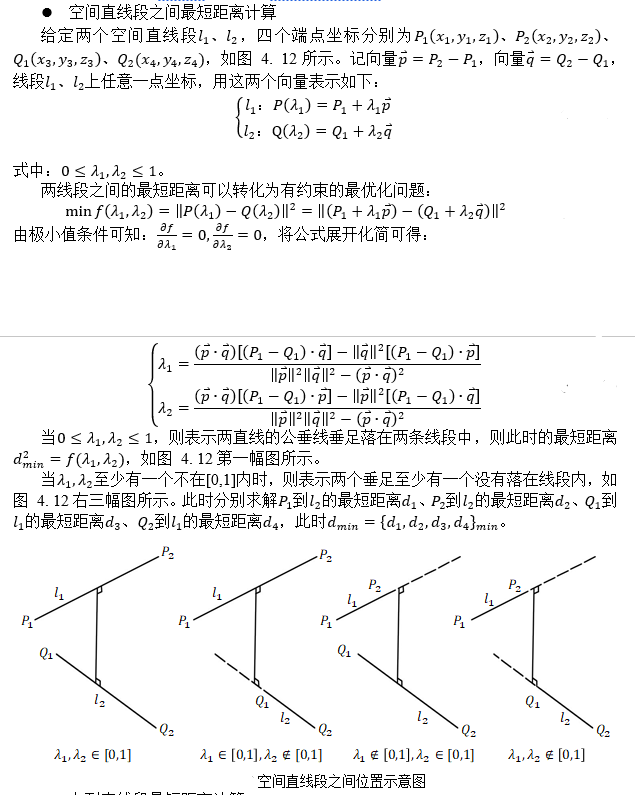点到直线段最短距离公式：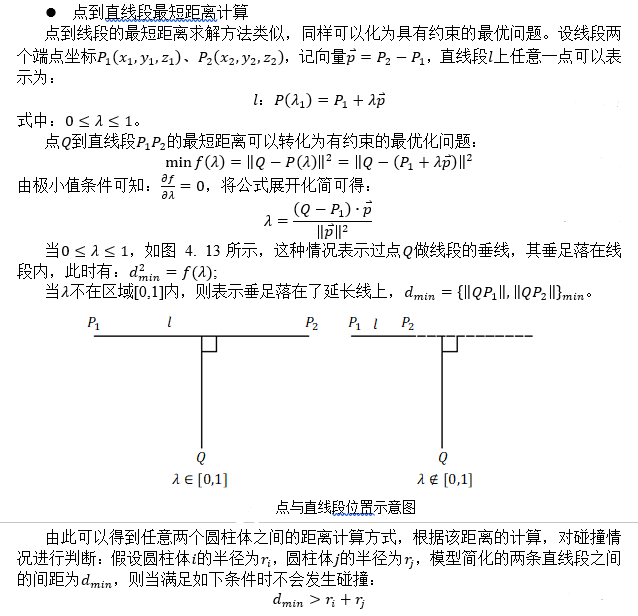%求空间中任意两条直线之间的最短距离，p1p2是直线段p的两个端点坐标，q1q2是直线段q的两个端点坐标
function [d]=distance(p1,p2,q1,q2)
s1=p2-p1;%方向向量
s2=q2-q1;

res1=((s1*s2')*((p1-q1)*s2')-(s2*s2')*((p1-q1)*s1'))/((s1*s1')*(s2*s2')-(s1*s2')*(s1*s2'));     %lamta1
res2=-((s1*s2')*((p1-q1)*s1')-(s1*s1')*((p1-q1)*s2'))/((s1*s1')*(s2*s2')-(s1*s2')*(s1*s2'));    %lamta2
if(res1<=1&&res1>=0&&res2<=1&&res2>=0)  %如果两个垂足都在两个直线段上，则将lamta带入，可得到两个垂足坐标，求其长度即可
tmp1=p1+res1*s1;
tmp2=q1+res2*s2;
tmp=tmp1-tmp2;
d=sqrt(tmp*tmp');
else
res3=(q1-p1)*s1'/(s1*s1');           %如果有垂足不落在线段上，需要进行下一步判断，分别计算两个线段的四个坐标点，到另外一条线段的长度
if (res3>=0&&res3<=1)                %q1点到p1p2线段的距离，同样计算lamta,如果在0~1表示垂足在线段上，带入求值
tmp=q1-(p1+res3*s1);
d1=sqrt(tmp*tmp');
else
d1=sqrt(min((q1-p1)*(q1-p1)',(q1-p2)*(q1-p2)'));%如果垂足不在线段上，直接判断到达两个端点的线段长度即可，取最小
end
res4=(q2-p1)*s1'/(s1*s1');           %q2到线段p1p2距离
if (res4>=0&&res4<=1)
tmp=q2-(p1+res4*s1);
d2=sqrt(tmp*tmp');
else
d2=sqrt(min((q2-p1)*(q2-p1)',(q2-p2)*(q2-p2)'));
end

res5=(p1-q1)*s2'/(s2*s2');            %p1到线段q1q2距离
if (res5>=0&&res5<=1)
tmp=p1-(q1+res5*s2);
d3=sqrt(tmp*tmp');
else
d3=sqrt(min((p1-q1)*(p1-q1)',(p1-q2)*(p1-q2)'));
end

res6=(p2-q1)*s2'/(s2*s2');            %p2到线段q1q2距离
if (res6>=0&&res6<=1)
tmp=p2-(q1+res6*s2);
d4=sqrt(tmp*tmp');
else
d4=sqrt(min((p2-q1)*(p2-q1)',(p2-q2)*(p2-q2)'));
end
d=min(min(d1,d2),min(d3,d4));%取四个最短的一个即可
end

end

展开全文matlab
• 知识都是环环相扣的, 在阅读这编文章之前, 要求懂两个知识点 1. 会求点到线段的最短距离 传送门 2. 会判断点与线段位置关系 传送门 如果上面两个知识点都懂, 那么就进入正题了 ...(1) 两线段相交成 X...

知识都是环环相扣的, 在阅读这编文章之前, 要求懂两个知识点

1. 会求点到线段的最短距离     传送门

2. 会判断点与线段位置关系     传送门

如果上面两个知识点都懂, 那么就进入正题了

给出点A1、A2的坐标, 构成线段A1A2, 再给出点B1,B2的坐标, 构成线段B1B2, 求线段A1A2与线段B1B2的最短距离

两条线段的摆放有很多情况

(1) 两线段相交成 X 型

(2) 两线段相交成 T 型

(3) 两线段相交成 ^ 型, 其中有两点重合

(4) 四个点在一条直线上, 视为相交

(5) 两线段不相交

判断两线段是否相交

(1)(2)(3)(4)是两条线段相交的例子, 对于两线段相交的情况, 距离为0, 只要对[判断点与线段位置关系]加以应用, 就能轻松判断出两线段是否相交。

分别检查两条线段, 如果双方都符合"另一条线段的两个端点分别位于当前线段的顺时针方向和逆时针方向", 则两条线段相交。

判断线段A1A2与线段B1B2是否相交的程序可以像下面这样写

if (dir(A1, A2, B1)*dir(A1, A2, B2) <= 0 && dir(B1, B2, A1)*dir(B1, B2, A2) <= 0)
cout << "相交" << endl;

只要事先将 dir 返回值定义为

逆时针             返回   -1

顺时针             返回    1

反向延长线上  返回    -2

延长线上          返回    2

线段上              返回    0

dir(A1,A2,B1)*dir(A1,A2,B2) 在B1、B2位于不同侧时就会得出 -1,B1或B2位于线段A1A2上时得出0。点A1、A2相对于线段B1B2的位置也是同理。接下来, 只要线段A1A2、B1B2的判断均小于等于0, 即可确定他们相交。

若不相交, 求两线段最短距离

在了解如何求点到线段的最短距离, 这就好办了

线段A1A2与线段B1B2的距离为以下四个距离中最小的一个

1.点A1到线段B1B2的距离

2.点A2到线段B1B2的距离

3.点B1到线段A1A2的距离

4.点B2到线段A1A2的距离

程序代码参考

#include<iostream>
#include<cmath>
#include<algorithm>
using namespace std;
typedef struct node
{
double x, y;
}Point, Vector;
double cross(Point A, Point B, Point P)      //向量AB与向量AP的外积（叉积）
{
Vector AB = { B.x - A.x, B.y - A.y };
Vector AP = { P.x - A.x, P.y - A.y };
return AB.x*AP.y - AB.y*AP.x;
}
double dot(Point A, Point B, Point P)         //向量AB与向量AP的内积（点积）
{
Vector AB = { B.x - A.x, B.y - A.y };
Vector AP = { P.x - A.x, P.y - A.y };
return AB.x*AP.x + AB.y*AP.y;
}
double dis2(Point a, Point b)                //点a、b距离的平方
{
return (a.x - b.x)*(a.x - b.x) + (a.y-b.y)*(a.y-b.y);
}
int dir(Point A, Point B, Point P)            //点P与线段AB位置关系
{
if (cross(A, B, P) < 0) return -1;     //逆时针
else if (cross(A, B, P)>0) return 1;   //顺时针
else if (dot(A, B, P) < 0) return -2;  //反延长线
else if (dot(A, B, P) >= 0&&dis2(A,B)>=dis2(A,P))
{
if (dis2(A, B) < dis2(A, P)) return 2;  //延长线
return 0;                          //在线上
}
}
double disMin(Point A, Point B, Point P)      //点P到线段AB的最短距离
{
double r = ((P.x-A.x)*(B.x-A.x) + (P.y-A.y)*(B.y-A.y)) / dis2(A, B);
if (r <= 0) return sqrt(dis2(A, P));
else if (r >= 1) return sqrt(dis2(B, P));
else
{
double AC = r*sqrt(dis2(A,B));
return sqrt(dis2(A,P)-AC*AC);
}
}
int main()
{
Point A1, A2, B1, B2;
cin >> A1.x >> A1.y;
cin >> A2.x >> A2.y;
cin >> B1.x >> B1.y;
cin >> B2.x >> B2.y;
if (dir(A1, A2, B1)*dir(A1, A2, B2) <= 0 && dir(B1, B2, A1)*dir(B1, B2, A2) <= 0)  //两线段相交, 距离为0
cout << 0 << endl;
else                                                   //如不相交, 则最短距离为每个端点到另一条线段距离的最小值
cout << min({disMin(A1, A2, B1), disMin(A1, A2, B2), disMin(B1, B2, A1),disMin(B1,B2,A2)}) << endl;
return 0;
}

参考书籍: 挑战程序设计竞赛2

展开全文数学几何 线段距离 二维
• import numpy as np import math import cv2 def cal_pt_distance(pt1, pt2): dist = math.sqrt(pow(pt1-pt2,2) + pow(pt1-pt2,2)) return dist font = cv2.FONT_HERSHEY_SIMPLEX ...opencv python
• 该函数实现了 Vladimir J. LUMELSKY，“线段之间距离的快速... 用于计算条线段之间的最短距离。 它也处理所有退化情况（当线平行时，一条线是一个点，线都是点）。 或者，该函数可以返回线段上最近的个点。matlab
• 我有个数据框。一个包含properties locations，另一个包含railway stations locations。属性数据框样本(原始数据框由约700行组成)：properties=pd.DataFrame({'propertyID':['13425','32535','43255','52521'],'...
• arcgis中求点与点、点与线之间的最短距离，路径分析，路径分析
• 点与线段_线段与线段的最短距离，matlab代码。自编的function，便于嵌入使用线段距离
•  通过线段端点，写成参数函数（考虑了斜率不存在的问题） 程序： function practice(points1，points2) g=points1(2)-points1(4); h=points1(1)-points1(3); if g&lt;0|h&lt;0 t1=-1:0...matlab
• 主要思路：假设有条曲线分别是c1和c2，把c1按照1的距离划分我这里用变量jd表示，得到一个曲线集合coll，然后遍历coll，得到coll中每一个曲线的个端点，再用这个端点分别求离曲线c2的最短距离，直接使用开发库...python
• 只回答定点到折线的最短距离的求法。一般由目测，即可看出哪一段折线是离定点最近的折线(例如你画的那个，显然DE就是离定点最近的折线段)，如看不出多算几段也可以，例如可以算每段折线离定点最近的点，则所有线段中...
• Mathematica程序 计算两线段之间的最短距离
• arcgis engine计算点到线最短距离 IProximityOperator接口用于获取个几何图形的距离，以及给定一个Point，求另一个几何图形上离离给定点最近的点。IProximityOperator接口的主要方法有：QueryNearesPoint,...
• 点到线段的最短距离

千次阅读 2019-08-29 09:40:05
点到线段的最短距离有三种情况： 最短距离分别是AP,CP,BP.第一种情况和第三种情况基本相似。首先我们要判断点在线段端点的两侧（1,3）还是在个端点之间（2）。假设p垂直于直线AB的交点为C，我们只需要计算出AC...
• // 点到直线的最短距离的判断 点（x0,y0） 到由点组成的线段（x1,y1） ,( x2,y2 ) private double pointToLine(int x1, int y1, int x2, int y2, int x0, int y0) { double space = 0; double a, b, c; a...java 线性代数
• 听起来好像你可以使用生成树算法 . 在伪代码中：build_tree(unconnected_nodes):connected_nodes = set()// pick a random unconnected nodeconnected_nodes.add(unconnected_nodes.pop())while not empty...java求最短距离
• 天做开发的时候，有个小伙伴问我怎么计算点到直线的最短距离，看样子困扰了他挺久，所以借这个机会吧这个问题记录下来，让更多小伙伴能涨一下姿势。 先看图：已知条件如下，p1、p2、target三个点，求红色线段的...unity unity3d 数学
• 目录0. 最短路径1. 准备工作数据准备（以湖北...打开属性表2.1.4 导出湖北省的边界2.1.5 导出地级市边界2.2 创建网络数据集2.2.1 创建文件数据库2.2.2 导入要素类2.2.3 创建网络数据集2.3 计算城市间网络距离2.3.1 打开
• 直线的信息可以以个端点的形式给出，也可以以一个直线上的点和直线的方向向量给出。本文中假设这条直线不...求直线的最短距离？ 直线l0我们可以用方程表示为：  （1） 直线段l1我们也可以用方程表示为：c++编程 最小二乘法 计算机图形学 直线
• 在双目视觉立体空间重建中，会根据个相机中...根据物体图像坐标、相机内参、给定坐标系的相机外参，求取相机光轴线的方程，从而实现立体重建，本文主要是解决在已知三维空间条直线求其最短距离、最近点及算法实现。opencv c++算法实现算法
• 点到直线的最短距离 ​ 根据直线的表示方式p = at + b，可以设一个点P(px,py)，任意一点A(x0,y0)到点P的距离可以根据点间距离公式求出。 D^2 = (px-x0)^2+(py-y0)^2 = (axt+bx-x0)^2+(ayt+by-y0)^2 = ...点到直线的最短距离
• 在我们只知道：如何计算点之间的距离直线上的一个点可以表示为点和一个参数的函数我们知道求函数的最小值(列表与代码块不是朋友)def distance(a, b):"""Calculate a distance between two points."""return n...
•算法 几何
• 一种点到线段的最短距离的方法 下面介绍点到线段的最短距离的方法： 图 1-6点到线段的最短距离 图1-6描述了一种计算点D到线段AC的最短距离的计算方法。直线方程由经过点A (x1,y1) 和 C (x2,y2)确定： B = A ...
• import java.io.IOException;import java.math.BigDecimal;import java.text.MessageFormat;public class LocationUtils {// 以下为 获得 点之间最短距离private static final BigDecimal EA...
• 计算点到线段的最短距离

热门讨论 2013-04-09 17:42:49
这个计算点到线段最短距离算法是先计算线段上距离点最近的点，然后求出 2...在计算用户到当前位置到某条公路的最短距离等实际情况中，可以先用这个算法，然后根据结果坐标使用 CLLocation 类的函数计算实际距离 VC6工程...